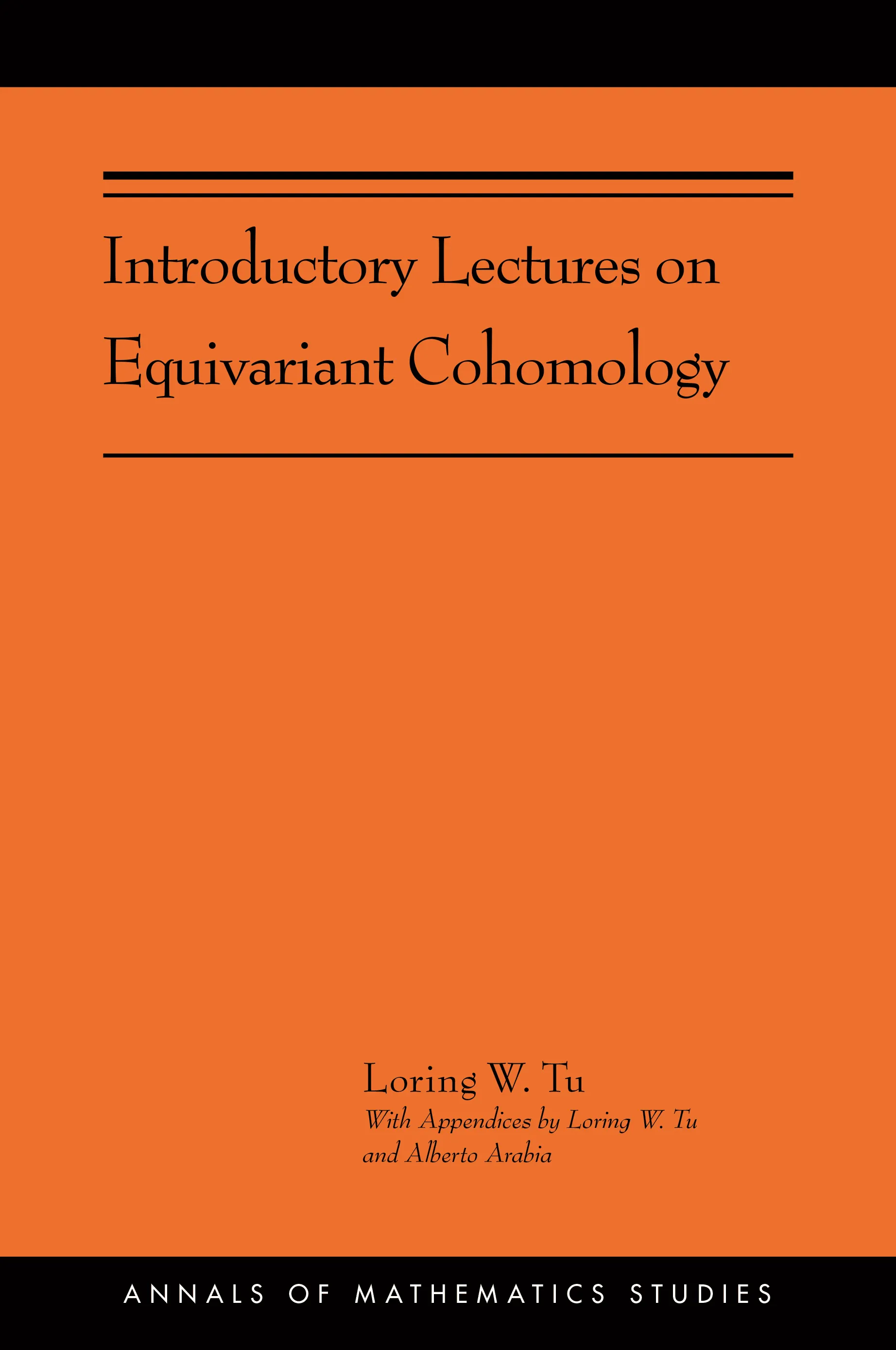#### Introductory Lectures on Equivariant Cohomology

Loring W. Tu

##### ebooks

Many of our ebooks are available for purchase from these online vendors:

Many of our ebooks are available through library electronic resources including these platforms:

# Introductory Lectures on Equivariant Cohomology: (AMS-204)## Paperback

Price:
\$75.00 / £62.00
ISBN:
Published:
Mar 3, 2020
2020
Pages:
200
Size:
6.13 x 9.25 in.
Illus:
37 b/w illus.

This book gives a clear introductory account of equivariant cohomology, a central topic in algebraic topology. Equivariant cohomology is concerned with the algebraic topology of spaces with a group action, or in other words, with symmetries of spaces. First defined in the 1950s, it has been introduced into K-theory and algebraic geometry, but it is in algebraic topology that the concepts are the most transparent and the proofs are the simplest. One of the most useful applications of equivariant cohomology is the equivariant localization theorem of Atiyah-Bott and Berline-Vergne, which converts the integral of an equivariant differential form into a finite sum over the fixed point set of the group action, providing a powerful tool for computing integrals over a manifold. Because integrals and symmetries are ubiquitous, equivariant cohomology has found applications in diverse areas of mathematics and physics.

Assuming readers have taken one semester of manifold theory and a year of algebraic topology, Loring Tu begins with the topological construction of equivariant cohomology, then develops the theory for smooth manifolds with the aid of differential forms. To keep the exposition simple, the equivariant localization theorem is proven only for a circle action. An appendix gives a proof of the equivariant de Rham theorem, demonstrating that equivariant cohomology can be computed using equivariant differential forms. Examples and calculations illustrate new concepts. Exercises include hints or solutions, making this book suitable for self-study.